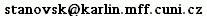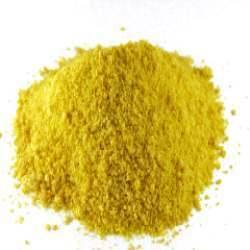H2WO4 (tungstic acid)

Description

Tungstic acid is a substance reacting with problems in the first order logic with special arithmetical functions (as defined in the TPTP library), producing a code in Wolfram's Mathematica that attempts to find a solution. The first version is a simple script translating between the two languages and calling built-in functions of Mathematica to solve the problem. Various combinations of the following three are used:

1. the Reduce and FullSimplify functions, for simplifying expressions - a problem is solved if simplified to True or False
2. the FindInstance function, for solving systems of equations over specified domain - an existential problem is solved if a solution is found or if no solutions exists

Implementation

Tungstic acid is a pair of Perl scripts: one for parsing TPTP problems into Mathematica, the other for processing Mathematica's output. They were tested with the text-based interface of Mathematica 7.0. The input is in the TFF0 syntax of the TPTP library.

Contact: